# VCE Unit 01 (2).pptx

20 de Apr de 2022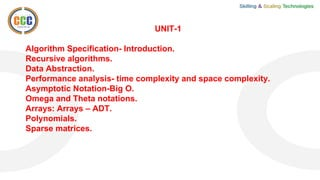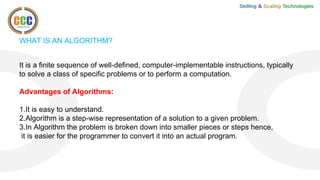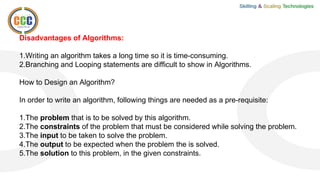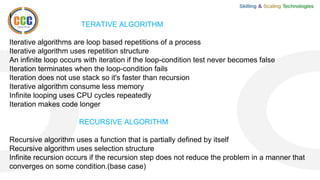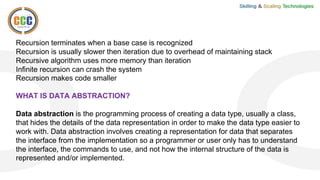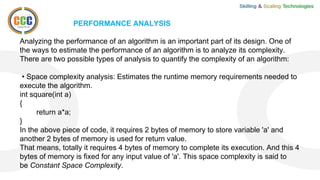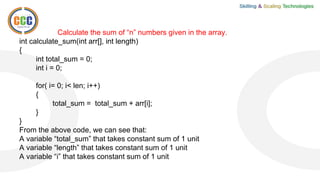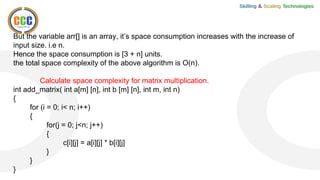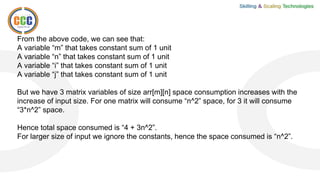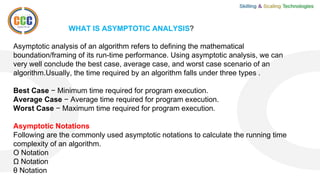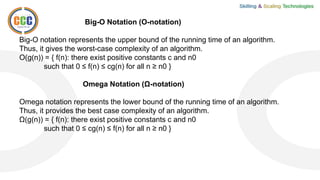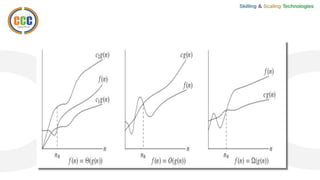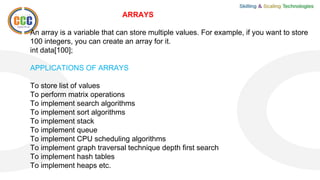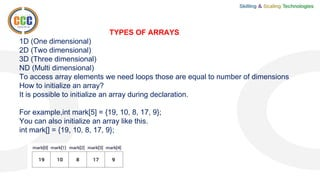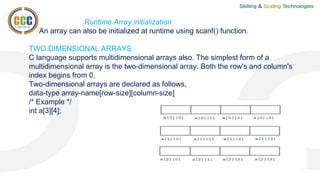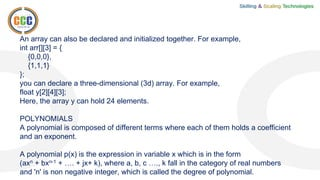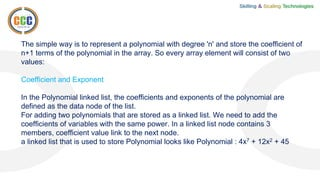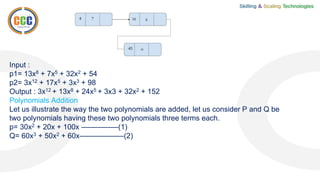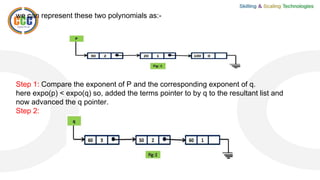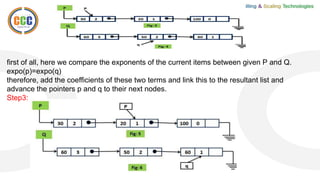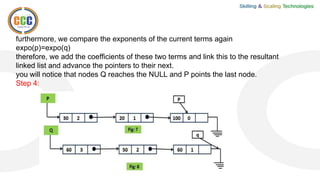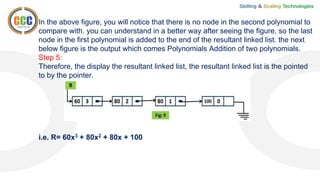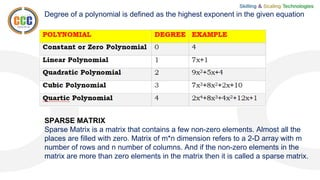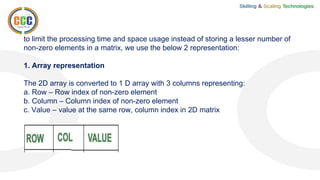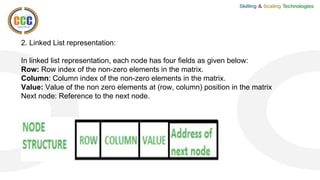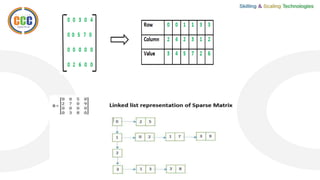1 de 28

### VCE Unit 01 (2).pptx

1. UNIT-1 Algorithm Specification- Introduction. Recursive algorithms. Data Abstraction. Performance analysis- time complexity and space complexity. Asymptotic Notation-Big O. Omega and Theta notations. Arrays: Arrays – ADT. Polynomials. Sparse matrices.
2. WHAT IS AN ALGORITHM? It is a finite sequence of well-defined, computer-implementable instructions, typically to solve a class of specific problems or to perform a computation. Advantages of Algorithms: 1.It is easy to understand. 2.Algorithm is a step-wise representation of a solution to a given problem. 3.In Algorithm the problem is broken down into smaller pieces or steps hence, it is easier for the programmer to convert it into an actual program.
3. Disadvantages of Algorithms: 1.Writing an algorithm takes a long time so it is time-consuming. 2.Branching and Looping statements are difficult to show in Algorithms. How to Design an Algorithm? In order to write an algorithm, following things are needed as a pre-requisite: 1.The problem that is to be solved by this algorithm. 2.The constraints of the problem that must be considered while solving the problem. 3.The input to be taken to solve the problem. 4.The output to be expected when the problem the is solved. 5.The solution to this problem, in the given constraints.
4. I TERATIVE ALGORITHM Iterative algorithms are loop based repetitions of a process Iterative algorithm uses repetition structure An infinite loop occurs with iteration if the loop-condition test never becomes false Iteration terminates when the loop-condition fails Iteration does not use stack so it's faster than recursion Iterative algorithm consume less memory Infinite looping uses CPU cycles repeatedly Iteration makes code longer RECURSIVE ALGORITHM Recursive algorithm uses a function that is partially defined by itself Recursive algorithm uses selection structure Infinite recursion occurs if the recursion step does not reduce the problem in a manner that converges on some condition.(base case)
5. Recursion terminates when a base case is recognized Recursion is usually slower then iteration due to overhead of maintaining stack Recursive algorithm uses more memory than iteration Infinite recursion can crash the system Recursion makes code smaller WHAT IS DATA ABSTRACTION? Data abstraction is the programming process of creating a data type, usually a class, that hides the details of the data representation in order to make the data type easier to work with. Data abstraction involves creating a representation for data that separates the interface from the implementation so a programmer or user only has to understand the interface, the commands to use, and not how the internal structure of the data is represented and/or implemented.
6. Smartphone Example An example in the real-world is a mobile or smartphone. The typical smartphone user does not know how calls are made, or how the phone accesses the Internet, or even how data is stored in the phone. Instead, the mobile or smartphone user is provided with an interface that hides these details from them. Making a call simply involves selecting a contact's phone number. Accessing the Internet involves selecting an icon on the screen. The user does not need to understand the details of how a phone call is placed or how the Internet works. These details are abstracted away from the phone's interface in order to make the phone easier and more effective to use. The design of the mobile or smartphone clearly separates the interface from the implementation. The user can learn the functions of the phone without ever having to know anything about how the phone works internally.
7. PERFORMANCE ANALYSIS Analyzing the performance of an algorithm is an important part of its design. One of the ways to estimate the performance of an algorithm is to analyze its complexity. There are two possible types of analysis to quantify the complexity of an algorithm: • Space complexity analysis: Estimates the runtime memory requirements needed to execute the algorithm. int square(int a) { return a*a; } In the above piece of code, it requires 2 bytes of memory to store variable 'a' and another 2 bytes of memory is used for return value. That means, totally it requires 4 bytes of memory to complete its execution. And this 4 bytes of memory is fixed for any input value of 'a'. This space complexity is said to be Constant Space Complexity.
8. Calculate the sum of “n” numbers given in the array. int calculate_sum(int arr[], int length) { int total_sum = 0; int i = 0; for( i= 0; i< len; i++) { total_sum = total_sum + arr[i]; } } From the above code, we can see that: A variable “total_sum” that takes constant sum of 1 unit A variable “length” that takes constant sum of 1 unit A variable “i” that takes constant sum of 1 unit
9. But the variable arr[] is an array, it’s space consumption increases with the increase of input size. i.e n. Hence the space consumption is [3 + n] units. the total space complexity of the above algorithm is O(n). Calculate space complexity for matrix multiplication. int add_matrix( int a[m] [n], int b [m] [n], int m, int n) { for (i = 0; i< n; i++) { for(j = 0; j<n; j++) { c[i][j] = a[i][j] * b[i][j] } } }
10. From the above code, we can see that: A variable “m” that takes constant sum of 1 unit A variable “n” that takes constant sum of 1 unit A variable “i” that takes constant sum of 1 unit A variable “j” that takes constant sum of 1 unit But we have 3 matrix variables of size arr[m][n] space consumption increases with the increase of input size. For one matrix will consume “n^2” space, for 3 it will consume “3*n^2” space. Hence total space consumed is “4 + 3n^2”. For larger size of input we ignore the constants, hence the space consumed is “n^2”.
11. WHAT IS ASYMPTOTIC ANALYSIS? Asymptotic analysis of an algorithm refers to defining the mathematical boundation/framing of its run-time performance. Using asymptotic analysis, we can very well conclude the best case, average case, and worst case scenario of an algorithm.Usually, the time required by an algorithm falls under three types . Best Case − Minimum time required for program execution. Average Case − Average time required for program execution. Worst Case − Maximum time required for program execution. Asymptotic Notations Following are the commonly used asymptotic notations to calculate the running time complexity of an algorithm. Ο Notation Ω Notation θ Notation
12. Big-O Notation (O-notation) Big-O notation represents the upper bound of the running time of an algorithm. Thus, it gives the worst-case complexity of an algorithm. O(g(n)) = { f(n): there exist positive constants c and n0 such that 0 ≤ f(n) ≤ cg(n) for all n ≥ n0 } Omega Notation (Ω-notation) Omega notation represents the lower bound of the running time of an algorithm. Thus, it provides the best case complexity of an algorithm. Ω(g(n)) = { f(n): there exist positive constants c and n0 such that 0 ≤ cg(n) ≤ f(n) for all n ≥ n0 }
13. ARRAYS An array is a variable that can store multiple values. For example, if you want to store 100 integers, you can create an array for it. int data; APPLICATIONS OF ARRAYS To store list of values To perform matrix operations To implement search algorithms To implement sort algorithms To implement stack To implement queue To implement CPU scheduling algorithms To implement graph traversal technique depth first search To implement hash tables To implement heaps etc.
14. TYPES OF ARRAYS 1D (One dimensional) 2D (Two dimensional) 3D (Three dimensional) ND (Multi dimensional) To access array elements we need loops those are equal to number of dimensions How to initialize an array? It is possible to initialize an array during declaration. For example,int mark = {19, 10, 8, 17, 9}; You can also initialize an array like this. int mark[] = {19, 10, 8, 17, 9};
15. Runtime Array initialization An array can also be initialized at runtime using scanf() function. TWO DIMENSIONAL ARRAYS C language supports multidimensional arrays also. The simplest form of a multidimensional array is the two-dimensional array. Both the row's and column's index begins from 0. Two-dimensional arrays are declared as follows, data-type array-name[row-size][column-size] /* Example */ int a;
16. An array can also be declared and initialized together. For example, int arr[] = { {0,0,0}, {1,1,1} }; you can declare a three-dimensional (3d) array. For example, float y; Here, the array y can hold 24 elements. POLYNOMIALS A polynomial is composed of different terms where each of them holds a coefficient and an exponent. A polynomial p(x) is the expression in variable x which is in the form (axn + bxn-1 + …. + jx+ k), where a, b, c …., k fall in the category of real numbers and 'n' is non negative integer, which is called the degree of polynomial.
17. The simple way is to represent a polynomial with degree 'n' and store the coefficient of n+1 terms of the polynomial in the array. So every array element will consist of two values: Coefficient and Exponent In the Polynomial linked list, the coefficients and exponents of the polynomial are defined as the data node of the list. For adding two polynomials that are stored as a linked list. We need to add the coefficients of variables with the same power. In a linked list node contains 3 members, coefficient value link to the next node. a linked list that is used to store Polynomial looks like Polynomial : 4x7 + 12x2 + 45
18. Input : p1= 13x8 + 7x5 + 32x2 + 54 p2= 3x12 + 17x5 + 3x3 + 98 Output : 3x12 + 13x8 + 24x5 + 3x3 + 32x2 + 152 Polynomials Addition Let us illustrate the way the two polynomials are added, let us consider P and Q be two polynomials having these two polynomials three terms each. p= 30x2 + 20x + 100x —————(1) Q= 60x3 + 50x2 + 60x——————(2)
19. we can represent these two polynomials as:- Step 1: Compare the exponent of P and the corresponding exponent of q. here expo(p) < expo(q) so, added the terms pointer to by q to the resultant list and now advanced the q pointer. Step 2:
20. first of all, here we compare the exponents of the current items between given P and Q. expo(p)=expo(q) therefore, add the coefficients of these two terms and link this to the resultant list and advance the pointers p and q to their next nodes. Step3:
21. furthermore, we compare the exponents of the current terms again expo(p)=expo(q) therefore, we add the coefficients of these two terms and link this to the resultant linked list and advance the pointers to their next. you will notice that nodes Q reaches the NULL and P points the last node. Step 4:
22. In the above figure, you will notice that there is no node in the second polynomial to compare with. you can understand in a better way after seeing the figure, so the last node in the first polynomial is added to the end of the resultant linked list. the next below figure is the output which comes Polynomials Addition of two polynomials. Step 5: Therefore, the display the resultant linked list, the resultant linked list is the pointed to by the pointer. i.e. R= 60x3 + 80x2 + 80x + 100
23. Degree of a polynomial is defined as the highest exponent in the given equation SPARSE MATRIX Sparse Matrix is a matrix that contains a few non-zero elements. Almost all the places are filled with zero. Matrix of m*n dimension refers to a 2-D array with m number of rows and n number of columns. And if the non-zero elements in the matrix are more than zero elements in the matrix then it is called a sparse matrix.
24. to limit the processing time and space usage instead of storing a lesser number of non-zero elements in a matrix, we use the below 2 representation: 1. Array representation The 2D array is converted to 1 D array with 3 columns representing: a. Row – Row index of non-zero element b. Column – Column index of non-zero element c. Value – value at the same row, column index in 2D matrix
25. 2. Linked List representation: In linked list representation, each node has four fields as given below: Row: Row index of the non-zero elements in the matrix. Column: Column index of the non-zero elements in the matrix. Value: Value of the non zero elements at (row, column) position in the matrix Next node: Reference to the next node.